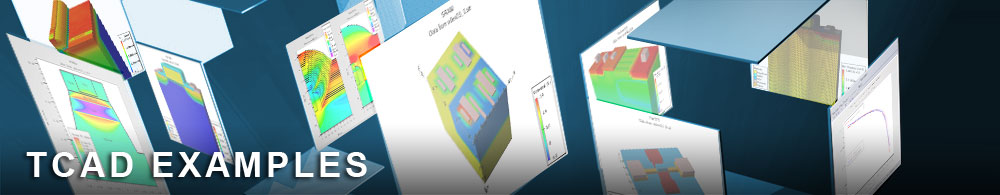Energy Balance and Drift Diffusion Comparison

## hemtex04.in : Energy Balance and Drift Diffusion Comparison

Requires: Blaze
Minimum Versions: Atlas 5.28.1.R

This example demonstrates Id-Vds calculations in single quantum-well AlGaAs/GaAs HEMT using Energy Balance (EB) and Drift Diffusion (DD) Models. It includes

• Construction of a heterojunction structure using Atlas syntax
• Material parameter specification
• Physical model specification including the energy balance model
• Simulation of Id-Vds characteristic with Vgs=0.0V
• Repeat simulation using drift-diffusion models for comparison
• Display of combined results in TonyPlot

The example file consists of two Atlas runs. Both use the same device structure defined in a similar manner to the previous two examples. The first run uses energy balance models to simulate Id-Vds characteristics and the second does the same electrical simulation using the classical drift-diffusion model. The aim of the example is to compare the effects of the different carrier transport models.

Energy balance models provide a more accurate description of physical device effects, in particular the effects of velocity overshoot and non-local impact ionization. These are not handled by the classical drift-diffusion model. These two effects can be observed in sub-micron HEMT simulation. This example concentrates on the velocity overshoot effect in the GaAs-AlGaAs structure. Non-local impact ionization effects can be seen in breakdown simulations. Since velocity overshoot is not accounted for in the drift-diffusion model, the results from simulations with this model will underestimate the current. This discrepancy gets worse as the channel length decreases.

The sequence of simulation syntax is similar to the previous example. For energy balance simulation, additional input parameters need to be defined in particular carrier energy and mobility relaxation times. In these examples the electron relaxation times taurel.el and taumob.el are defined in the two separate material statements for the GaAs and AlGaAs materials, overriding the default values. In the same statements the low field electron mobilities are specified for GaAs and AlGaAs. The saturation velocity is also explicitly defined here for GaAs. The material statement for AlGaAs also contains the align parameter, which defines the portion of the energy band gap difference going to the conduction band (60% in this example) at the GaAs-AlGaAs heterojunction. If the align parameter is present in the material statement the energy band alignment is calculated based on the value of this parameter rather than using electron affinities of the materials forming the respective heterojunction.

Since HEMTs are majority carrier devices and the impact-ionization and breakdown are not a subject of analysis here, the one carrier (electrons only) transport model is used in both continuity and energy balance equations. Thus the following three basic equations are solved in this example to simulate the device characteristics: Poisson, electron continuity and energy balance equations.

In the model statements the set of physical models used is specified: field dependent mobility, and the one carrier transport model (carriers=1 electron). The solution of the energy balance equation for electrons is activated in this statement using the parameter hcte.el.

The contact statement is used to specify the workfunction of the gate electrode, giving the Schottky barrier height of approximately 0.8V.

After the initial solution the gate voltage is set to 0, and the Id-Vds characteristic is calculated. First the drain voltage is ramped up to 0.1V. The solutions are obtained using the combined Gummel-Newton block algorithm specified in the following statement:

method gummel block newton

The algorithm implies that, if the solution does not converge in the course of Gummel iterations, the program will automatically switch over to the block algorithm where the Poisson and electron continuity equations are solved by the Newton algorithm with the frozen electron temperature, and then the electron continuity equation and energy balance equation are solved by the Newton algorithm. If the solution still does not converge, the program will switch over to the fully coupled Newton method.

Next the drain voltage is ramped up to 3V. This part of simulation is performed using the Newton method:

method newton

The results of the simulation are saved in a log file to be displayed later with the results of the DD simulation.

The second Atlas run repeats the first but uses the conventional one carrier Drift Diffusion Model: Poisson's equation and the electron continuity equation are solved self-consistently. The same set of physical models is used, except the energy balance equation. The latter is turned off by not indicating the hcte.el parameter in the models statement. The resulting Id-Vds characteristic is saved in a log file. The statement output con.band val.band e.velocity is used in both runs to save the conduction and valence band potentials and the electron velocity information to the solution files. Generating the solution files in the energy balance simulation and selecting to plot them will show the velocity overshoot effect.

Results from two runs with the EB and DD models are compared by overlaying the two log files in TonyPlot.

To load and run this example, select the Load button in DeckBuild > Examples. This will copy the input file and any support files to your current working directory. Select the Run button in DeckBuild to execute the example.